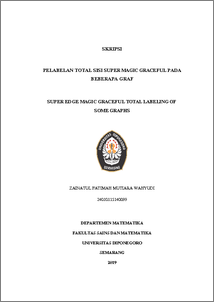# SUPER EDGE MAGIC GRACEFUL TOTAL LABELING OF SOME GRAPHS

Wahyudi, Zainatul Fatimah Mutiara (2019) SUPER EDGE MAGIC GRACEFUL TOTAL LABELING OF SOME GRAPHS. Undergraduate thesis, UNDIP.Preview
PDF
166KbPDF
Restricted to Repository staff only

1153KbPreview
PDF
27Kb

## Abstract

Let a ( , ) p q graph G with the vertex set V G( ) , the edge set E G( ) , the number of vertices is p, and the number of edges is q. The edge magic graceful total labeling of graph G is a bijection from V G E G ( ) ( )  to the integers {1, 2,..., } p q + such that for all edges, the absolute value of the sum of two adjacent vertex labels minus the edge label that connect the two vertexs is a constant k. The super edge magic labeling is edge magic labeling whose the set of vertex labels is {1, 2,..., }p . This final paper shows super edge magic graceful total labeling on path graph ( ) P n , cycle graph ( ) Cn , crown graph ( ) Cn + , and subdivided bistar graph 1, 1, : K K m n . Keywords : Edge magic graceful labeling, super edge magic graceful labeling, path graph, cycle graph, crown graph, subdivided bistar graph.

Item Type: Thesis (Undergraduate) Q Science > QA Mathematics Faculty of Science and Mathematics > Department of Mathematics 84223 INVALID USER 11 Jun 2022 19:11 11 Jun 2022 19:11

Repository Staff Only: item control page